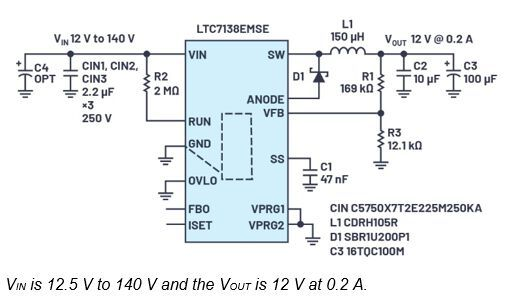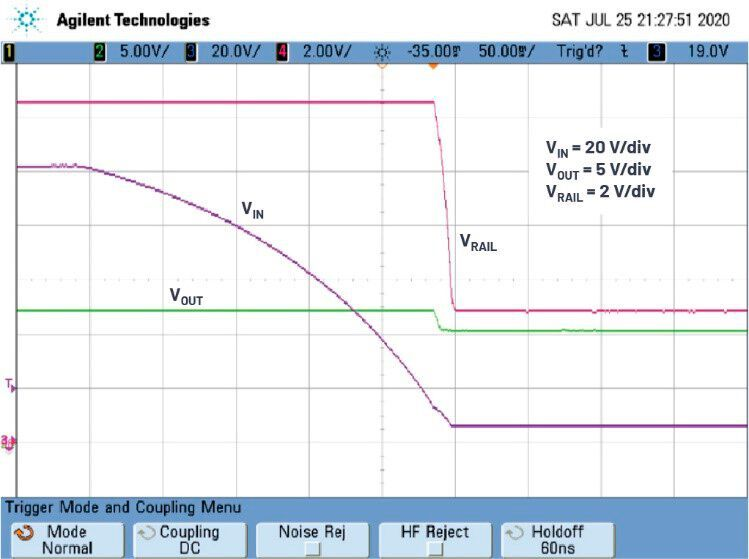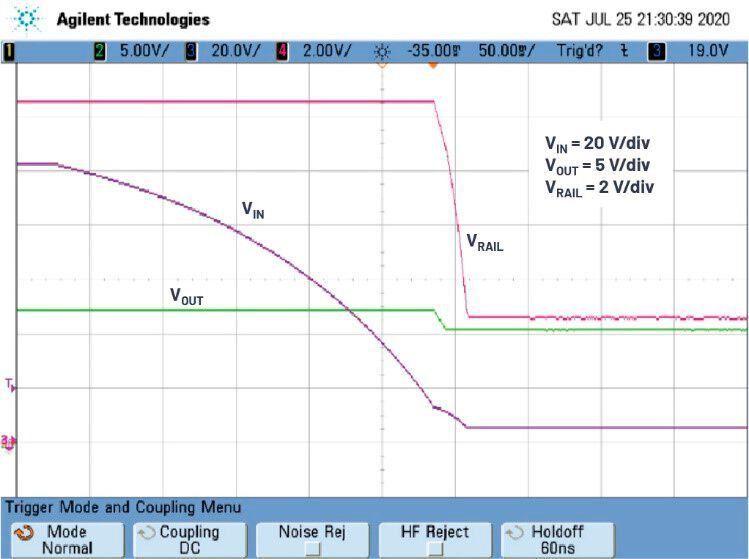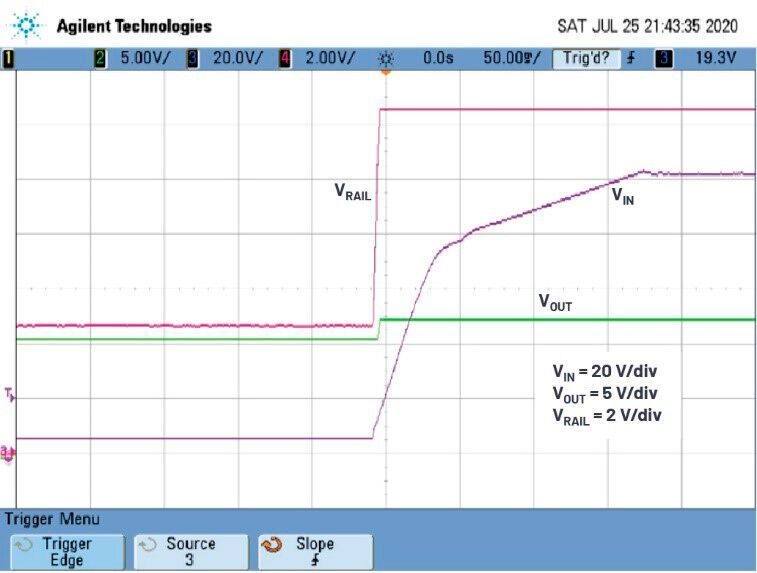## POWER SUPPLYWide 5 V to 140 V input to 12 V, 200 mA bias power supply

From Victor Khasiev

Related Vendorsless

Modern automotive and industrial systems require a stable voltage source even as the system input voltage sweeps from one extreme to another. This article describes solutions for maintaining bias voltages in electrical systems over a wide range of source voltages, from 5 V to 140 V.It’s important to keep major power systems operational over a wide input voltage range. This article discusses solutions to this goal. (Source: Olivier Le Moal)

In automotive systems, significant rail voltage variations can result from cold cranking startup, cylinder deactivation/activation in dynamic fuel management systems, or significant engine load changes. Likewise, in industrial applications, line brownouts are a problem, and the switching on of motors in high power equipment can lead to serious drops of input voltage.

Even when power conversion systems cannot provide full power to the loads at low voltage inputs, many of these systems must remain operational, regardless of the input voltage level. For example, widely used high voltage boost and buck converters employ high voltage MOSFETs with a standard gate level. At input dropout, the bias voltage should stay above the 10 V to keep the gate drivers operational. Critical digital control and information systems should be biased and functional regardless of the input conditions as well.

### Circuit description and functionality

If the input voltage is not expected to fall below the desirable bias level and the goal of design is to have an external bias power supply to minimize the power dissipation of the switching controllers, then a straightforward buck converter can be employed.

This approach is illustrated in Figure 1. The solution is centered around a high voltage buck-controller LTC7138 with internal switching transistors. The power train also includes inductor L1, diode D1, and output capacitors C2 and C3. To minimize the solution profile, to below 3 mm, only ceramic capacitors were used in the input. An optional polarized capacitor can be used as well (for example, a cost-effective 22 µF 200 V, EMVE201 ARA220MKG5S), but it considerably increases the height of the bias power supply.Figure 1. High voltage, buck-based bias circuit electrical schematic. (Source: Analog Devices)

This circuit was verified and tested, with the waveforms illustrating the functionality of the circuit presented in Figure 2. The initial input voltage level of 100 V drops to 12 V, but the output provides 0.2 A of the stable 12 V voltage to the load.

The performance outlook of this design significantly changes if the input voltage drops below the desired bias level. In this case, using just the buck converter is not enough, because the output voltage follows the input when it falls below the desired output. Figure 3 illustrates a solution to this problem using a dual-stage bias power supply. The first, main stage is a high voltage step-down converter similar to what is shown in Figure 1. It is output connected to a boost converter and based on an LT8330 converter IC with integrated power transistor. The power train includes inductor L2, diode D2, and an output filter. The voltage stress on the components in the boost converter circuit is much lower compared to the buck front end, which permits selection of relatively inexpensive parts and reduces the overall cost.

The output of the buck converter in this circuit is set to 12.5 V. However, the output of the boost converter is set to a lower voltage of 10.5 V, enough for the load to function properly. The converters are never operational at the same time. If one is switching, the second is not. In normal operating conditions (VIN > 12.5 V), when the input voltage changes from 12.5 V to 100 V, only the buck converter is active, providing 12.5 V to the load. The current flows to the load terminal VOUT through the inductor and diode of the boost converter. Due to relatively low current levels, the losses in this current path are minimal.

As long as VIN > 12.5 V, the voltage on the output of the boost converter is 12.5 V and by far exceeds the preset value of 10.5 V, so there’s no switching action in the boost section and only the buck is active.
As the input voltage reduces to the level of 12.5 V or below, the buck converter stops switching, but it keeps the internal P-channel MOSFET in the ON state, allowing 100 % duty cycle operation.
If input voltage falls below 12.5 V, then both voltages, VRAIL (intermediate rail) and VOUT, fall to the VIN level. In the 10.5 V < VRAIL < 12.5 V range of the intermediate rail, both the converter’s buck and boost do not switch.
If the input voltage continues to fall and the VRAIL level drops below 10.5 V, the boost converter becomes operational, keeping VOUT at 10.5 V.

The waveforms illustrating the functionality of this converter are presented in Figure 4. The minimum input voltage of 5.5 V at the load current is 0.15 A. Reducing the load to 0.1 A corresponds to a minimum input voltage of 5.0 V, as shown in Figure 5. The rise of the input voltage from 5 V to 100 V is illustrated in Figure 6. A photo of the converter is shown in Figure 7.

### Subscribe to the newsletter now

#### Don't Miss out on Our Best Content

Unfold for details of your consentFigure 4. High voltage, dual stage-based bias circuit waveforms. The load current is 0.15 A and the time scale is 50 ms/div. (Source: Analog Devices)Figure 5. High voltage, dual stage-based bias circuit waveforms. The load current is 0.1 A and the time scale is 50 ms/div. (Source: Analog Devices)Figure 6. The input voltage rises waveforms. The load current is 0.1 A and the time scale is 50 ms/div. (Source: Analog Devices)